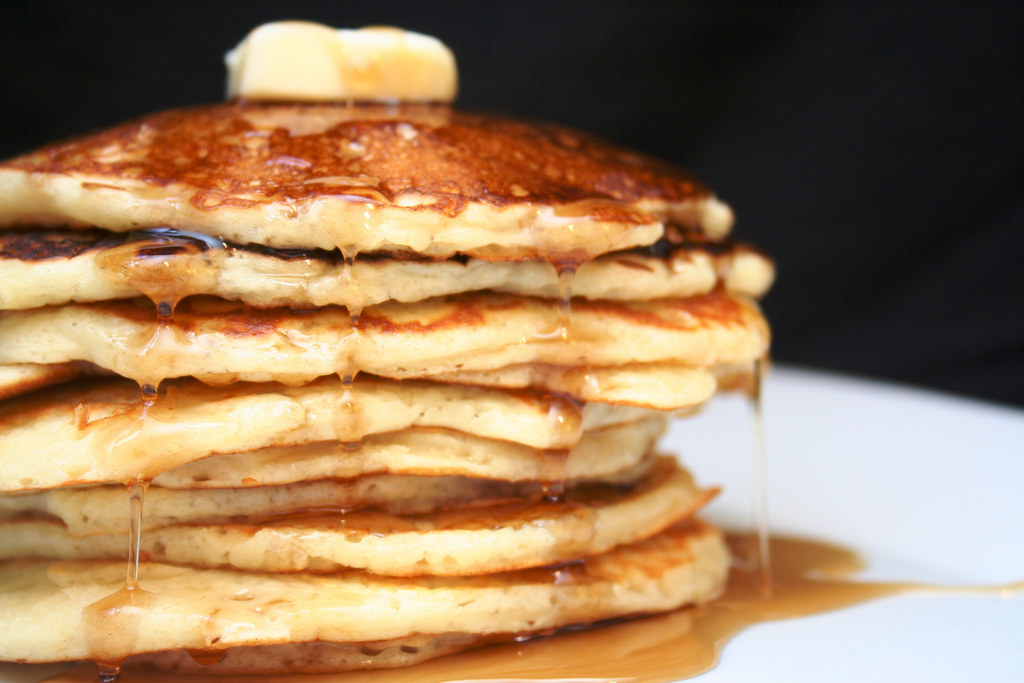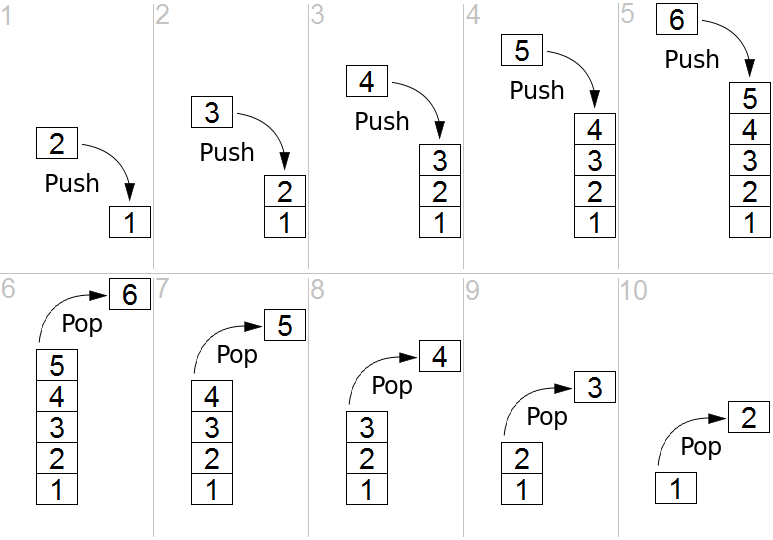## Stack

• a stack is a metaphor for a physical stack(like this yummy stack of pancakes)

## What makes a stack a stack

• a stack is an abstract data type with only two operators
• push adds an item to the top
• pop removes an item from the top
• a stack is a LIFO (last in, first out) structure
• you take things off the top of the stack in the reverse order from which you put them on
• contrast with a queue, which is FIFO (first in, first out)

## Pushing and Popping Visualized

Imagine a stack that starts with a single pancake ("1")(image source: https://en.wikipedia.org/wiki/Stack_(abstract_data_type), public domain)

## The Freedom of Constraints

Why only two operators?

• it is powerful because of its limitations
• easier to optimize
• easier to debug
• easier to understand code that uses it

The theme of "Freedom of Constraints" is important in software design.

(Also in any design or engineering context. And anything creative or artistic.)

## Arrays vs. Stacks

In JavaScript, the easiest way to implement a stack is by using an array.

In fact, every array already knows how to `push` and `pop`.

Try this in a JavaScript console:

``````let fruits = []
fruits.push("apple")
fruits.push("banana")
fruits                    // [ 'apple', 'banana' ]
fruits.push("cherry")
fruits                    // [ 'apple', 'banana', 'cherry' ]
let fruit = fruits.pop()
fruit                     // 'cherry'
fruits                    // [ 'apple', 'banana' ]
``````

Note that after a `pop`, the stack's contents are changed. Pop removes and returns the final value from the array.

## Stack Trace

You may have heard the term "stack trace". A stack trace is part of most error messages, e.g.:

``````ReferenceError: fizz is not defined
at Object.onceWrapper (events.js:254:19)
``````

## Stack Trace Explained

In this context the term "stack" refers to the call stack.

The JavaScript interpreter is a program, and that program uses a stack internally to keep track of the list of functions that call functions that call functions that call...

For instance, in the above stack trace, you can see that the function `TTY.onread` called the function `ReadStream.Readable.push`, which called the function `readableAddChunk`, and so on.

Now you know why a stack trace is upside down!

## Lab: Fibonacci Stack

Using a stack, put the following program into a file called `fib.js`...

``````let series = [0, 1];
while (series.length < 10) {

}
console.log(series);
``````

...and complete it so that running `node fib.js` prints

``````[ 0, 1, 1, 2, 3, 5, 8, 13, 21, 34 ]
``````

which are the first 10 elements of the Fibonacci sequence.

Although `series` is an array, please treat it like a stack -- that is, you can only use `series.push()` and `series.pop()`, not any other array operations.

Please split into pairs and do this right now. A solution is on the next slide.

## Solution: Fibonacci Stack

Hint
You will need to push the first two numbers you popped off in the opisite order you poped them ```js let a = series.pop() let b = series.pop() //...... series.push(b) series.push(a) ```
Solution
```js let series = [0, 1]; while (series.length < 10) { let b = series.pop(); let a = series.pop(); let c = a + b; series.push(a); series.push(b); series.push(c); } console.log(series); ```

## Uses for stacks

Stacks are useful in many scenarios